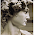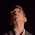### Just Me

Western music is out of tune. There's good reasons for this, if you want to play music on mechanical instruments and don't want to spend hours retuning them every time you change key. If, however, you don't need to change key, or you're using synthesisers that can retune in an instant, the only reason to use the western system is habit.

A musical scale is an ordered set of notes with mathematically related frequencies. Usually a scale is split into equal sized blocks, and the lowst note of each block is double the frequency of the lowest note of the block below. In almost all the music you hear in the western world, there are 12 notes in each block - confusingly called an octave - and each frequency is 2^(n/12) where n is the place of each note in the block, starting at 0.

This system is the result of centuries of compromise between mathematics, the practicalities of instrument manufacture, and fashions followed by composers and performers. There don't need to be 12 notes in a block, and their frequencies don't have to be related in this way.

Other systems are possible. One common alternative has five notes to each octave, where the frequency of the 2nd note is one and one eigth times the frequency of the first, the third 1+(1/4) times the first, the fourth 1+(1/3), the fifth 1+(2/3), and the first note of the octave above twice the one below.

Here's a slightly mad hypothetical 11 note octave:
Note 1: Frequency is f, where f=500
Note 2: f*(1+(1/7)) = f*(8/7) = 500*1.4286 = 571.42857
Note 3: f*(1+1/4)) = f*(5/4)= 500*1.25 = 625
Note 4: f*(1+(2/7)) = f*(9/7) = 500*1.28571 = 642.85714
Note 5: f*(1+(3/7)) = f*(10/7) = 500*1.42857 = 714.28571
Note 6: f*(1+(2/4)) = f*(6/4) = 500*.5 = 750
Note 7: f*(1+(4/7)) = f*(11/7) = 500*1.57143 = 785.71429
Note 8: f*(1+(5/7)) = f*(12/7) = 500*1.71429= 857.14286
Note 9: f*(1+(3/4)) = f*(7/4) = 500*1.75 = 875
Note 10: f*(1+(6/7)) = f*(13/7) = 500*1.85714 = 928.57143
Note 11: f*1+(7/7)) = f*(14/7) = 500*2 = 1000

I say it's mad, but it's quite usable, if a little strange-sounding to our ears. It's based on simple integer fractions (in this case with 7 and 4 as the denominators), just like most musical scales.

When we hear two simultainious frequencies, they sound pleasant together if their frequencies have a simple frational mathematical relation - if one is one third of the other, or two fifths, or three quarters, or something similar. Of course, the simplest relation (apart from the two frequencies being identical) is where one is half or double the other.

But, the standard western system isn't like that. f*2^(n/12) is a progression of fractional indices, not of fractions. So why do we use it?

The answer is that, if you're playing a tune in A Major, where the A note is 440Hz and the D note above it is one and a quarter times A (i.e 586.66Hz), then so long as you stay in A Major, your chords and harmonies will be sweet. But then you change to C Major, where C is tuned to 5/4 times A (making C 528Hz), and D is 9/8 times C. Unfortunately, the correct value for D in the key of C is now 594Hz.

But your instrument is still tuned to A Major, where D is 586Hz. It's 8Hz out - not a vast amount, but noticible, and the difference doubles if you go up an octave. Plus, the difference gets larger as the relative keys get more distant.

One solution to this probem is simply not to change keys - but that is quite a sacrifice to make. Another is to have several pianos onstage with you, all tuned to different keys - but that requires a big stage and a bigger bank account. You could make sure that when you change keys, you change to ones that won't be too out of tune - but that's a compromise that pleases no one.

A different approach is to make all keys slightly out of tune, but in such a way that none (or few) of them will go much more out of tune when you change keys. This means you can change key in the middle of a concert without offending your listener's ears too much - though they'll be slightly offended all the time.

There are several such systems, but the one which allows for any key change, each with equal dischordance, and which distorts all keys equally, is called Equal Temperament or Equal Tuning, and it's the one based on f*2^(n/12).

However, with music made using computers, there's no need to stick with Equal Temperament, and the only reason we generally do so it habit. The only great difficulties in adopting a 15 note octave are shoehorning them into 12 note midi keyboards and persuading your sequencer to be more broadminded. If you don't mind sticking with 12 notes it's not hard to use software to make the intervals 'correct'.

There is, though, one small issue with tuning your synthesiser to Just Temperament, as it's called. There are many possible Just Temperaments - many systems of fractional note relationships that could be used.

Even if we stay with the 17th century Just system which Equal Temperament approximates, the Minor Second interval (between the root note and the semitone above) could equally well be 16/15 (1.066) or 17/16 (1.062). The Minor Seventh interval (10 semitones up from the root) is sometimes given as 9/5 (1.8), sometimes 7/4 (1.75) or 16/9 (1.77). All three fractions give almost the same result, but not quite, and they're all equally viable.

I favour the system below, because it keeps the numbers low, and keeps them simply related to each other:

Note 1:f*(1/1) = 440*1=440
Note 2: f*(17/16) = 440*1.0625=467.50000
Note 3: f*(9/8) = 440*1.125=495
Note 4: f*( 6/5) = 440*1.2=528
Note 5: f*(5/4) = 440*1.25=550
Note 6: f*(4/3) = 440*1.33333=586.66667
Note 7: f*(7/5) = 440*1.4=616
Note 8: f*(3/2) = 440*1.5=660
Note 9: f*(8/5) = 440*1.6=704
Note 10: f*(5/3) = 440*1.66667=733.33333
Note 11: f*(7/4) = 440*1.75=770
Note 12: f*(9/5) = 440*1.8=792
Note 13: f*(2/1) = 440*2=880

1.I'm hereby challenging you to post about yourself. (Take a look at my place!) I hope you won't let me down. :-)

2.Hmmm. I'm about to spend the evening and night with my boyfriend, with no access to computers, so that gives me a few hours to ask myself the great question: Who Am I?

In other circumstances, this is usually accompanied by What Am I Doing?, Why Am I Doing It?, and When Can I Do Something More Interesting?

I'll post an answer as soon as I have one, and a computer in front of me.

Short answer in the meantime: I'm a British eccentric.

3.No special hurry has been requested. Moreover, beimg with «C» gets top priority, goes without saying. Enjoy it, you both!
As to your last sentence, great news travel fast, I'd say...